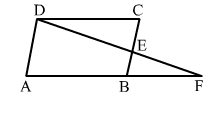# In the adjoining figure, ABCD is a parallelogram and E is the midpoint of side BC.

Question:

In the adjoining figure, ABCD is a parallelogram and E is the midpoint of side BC. If DE and AB when produced meet at F, prove that AF = 2ABSolution:

Given: ABCD is a parallelogram.
BE = CE    (E is the mid point of BC)
DE and AB when produced meet at F.
To prove: AF = 2AB

Proof:
In parallelogram ABCD, we have:
AB || DC
∠DCE = ∠EBF            (Alternate interior angles)
In ∆DCE and ∆BFE, we have:
∠DCE = ∠EBF              (Proved above)

∠DEC = ∠BEF              (Vertically opposite angles)
Also, BE = CE           (Given)
∴ ∆DCE ≅​ ∆BFE  (By ASA congruence rule)
∴ DC = BF         (CPCT)
But DC = AB, as ABCD is a parallelogram.
∴ DC = AB =  BF                   ...(i)

Now, AF = AB + BF                ...(ii)
From (i), we get:
AF = AB + AB = 2AB
Hence, proved.